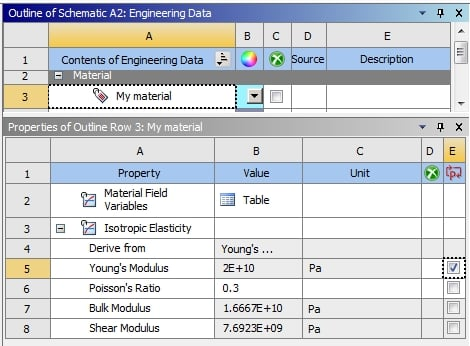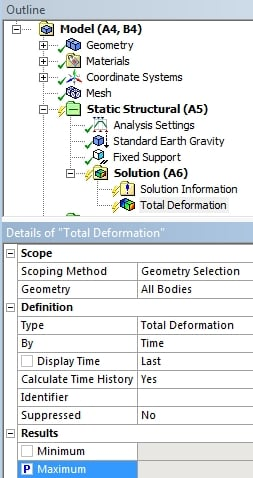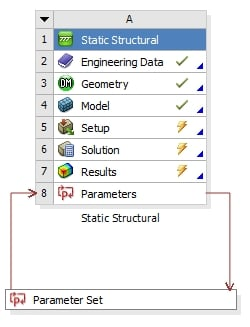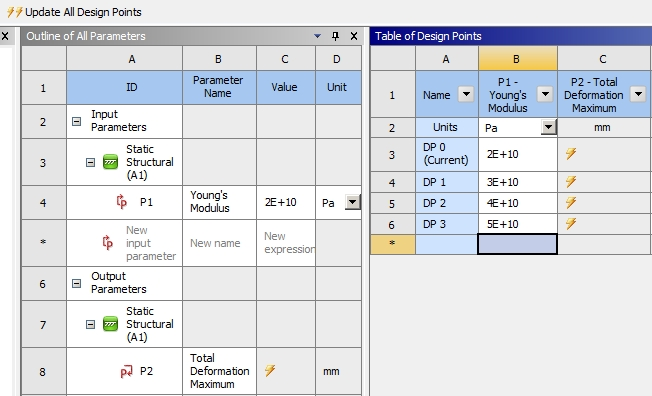## General Mechanical

•joepa_2017
Subscriber

How can I run multiple simulations on the same model with variable mechanical properties?

For example, let's say I have a rod that I am applying a tensile load to. I want to simulate the given load with multiple different values for the stiffness to look at how the results change with different values of stiffness. Lately, I have been creating new Workbench projects each time I change the stiffness so that I can view results for each set of data. But, is there a better way to do this?

That is, is there a way to input a set of stiffness values? Then, ANSYS will do the simulation for each stiffness value while still allowing me to plot the results for each stiffness value?

•peteroznewman
Subscriber

This is how to automate a parameter study

In Engineering Data, click the checkbox after Young's Modulus to turn it into a Parameter.If you have a Static Structural analysis and you have a Deformation result, you click the P box next to the Maximum.Then on the Project Schematic, you double click on the Parameter Set boxThen in the Parameter Set, you type a set of values for Young's Modulus, then click Update All Design Points [Corrected].ANSYS will go off and calculate a result for each value of Young's Modulus and put the Total Deformation Maximum into column C.

You can do the same with geometric shape parameters as shown in this video.

Regards,
Peter

•joepa_2017
Subscriber

Ok, thanks for all of the details! I was having a hard time finding help for using parameters. So, just to be clear, I can only extract things like maximum and minimum strain values for each value of stiffness? There's no way to see a stress distribution at each stiffness value using a parameters set?

It's ok if there isn't as I am still able to do that using my slower, manual method, and the max/min values would still be useful to get using parameters. It would just be incredibly convenient to get the entire solution set for strain at each node using a parameters set.

•peteroznewman
Subscriber

Yes you can have a complete solution for each row of the Parameter set. Past column C where the Deformation result will be put is a column titled Retain. If you check of every row, then the full solution will be retained and you can call each one up using the Set Current when you RMB on a row.

If my post above answered your question, please click Is Solution below that post to help others and mark this topic as Solved.

Regards,
Peter

•joepa_2017
Subscriber

I apologize for asking another question on this thread. The only thing I can't find now is where I can click Evaluate All Design Points. Is that within the Parameter Set?

•peteroznewman
Subscriber

Sorry, I meant to say Update All Design Points, which is the button on the top left of the image above, so I edited the original, thanks.